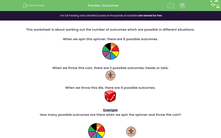# Calculate the Number of Outcomes

In this worksheet, students will work out how many different outcomes are possible in each situation.Key stage:  KS 3

Curriculum topic:   Probability

Curriculum subtopic:   Understand Probability Outcomes

Popular topics:   Probability worksheets

Difficulty level:#### Worksheet Overview

This activity is about working out the number of outcomes that are possible in different situations.

When we spin this spinner, there are 8 possible outcomes.When we throw this coin, there are 2 possible outcomes, heads or tails.When we throw this die, there are 6 possible outcomes.Example

How many possible outcomes are there when we spin the spinner and throw the coin?For each colour, we can get heads or we can get tails.

The number of outcomes = 8 x 2 = 16

In this activity, we are going to investigate possible outcomes from more than one event.### What is EdPlace?

We're your National Curriculum aligned online education content provider helping each child succeed in English, maths and science from year 1 to GCSE. With an EdPlace account you’ll be able to track and measure progress, helping each child achieve their best. We build confidence and attainment by personalising each child’s learning at a level that suits them.

Get started